## Arc Properties:

The manner in which the are medium is made to conduct has already been studied. A plot of instantaneous values of voltage eB between the electrodes of a burning arc against the corresponding values of current gives the Arc Properties. With the increase of arc current the temperature rises and the process of ionization becomes more active,: thereby increasing the conductivity of the medium and thus the arc voltage decreases. The increase of current in the higher range does increase the conductivity but has much less marked effect upon the voltage. The initial breakdown of the gap between the electrodes requires a high ignition voltage eZ at zero current (Fig. (13.3)). It is also seen that by changing the rate of change of current the characteristics shift. If the current changes rapidly with time the characteristics are known as dynamic and if the rate of change of current is small the characteristics are known as stalk. However, during most of the half cycle the quantity (di/dt)/i is small and a plot of voltage against current gives static characteristics. Near current zero when (di/dt)/i becomes large this will no longer be true.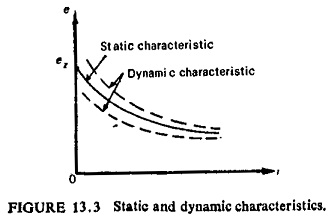If the current through the arc is suddenly changed it is found that the voltage across it does not assume the value corresponding to the new value of the current on the static characteristics. Thus if the current is suddenly increased the instantaneous voltage across it is found to be higher than that given by the static characteristics. The reason is that at any given current the arc and its surroundings have a heat content represented by the heated, dissociated, ionized gas which makes up the Arc Properties.

The total heat content in the steady state as well as the heat loss obviously depend on the current, but it takes time for this heat content to change and things depart therefore from the static characteristic until the new heat content is established. This is sometimes expressed by saying that there is thermal hysterisis in the changing arc.

When the current falls from a sufficiently high value, the transition is. entirely an effect of temperature. This means that the transition with falling temperature may take place at various instantaneous current values depending upon the rate of current fall that is, the thermal hysterisis effect must be considered. Thus, with rapidly-falling current the value at which the transition occurs may be much smaller than with slowly-falling current. This is one reason why such transitions are not generally observed in high current a.c. circuit breakers. Also in air-blast breakers the core temperature of the arc tends to be maintained at a high value right up to the current zero instant; and the possibility of transition becomes remote. Only in some oil circuit breakers, the efficient cooling of the arc because of the high thermal conductivity of the gas formed, enables the arc temperature to fall relatively quickly as the current decreases, and a transition to the high voltage takes place before current zero. The distribution of voltage along the arc is not linear. It is known that very close to the cathode there is a positive space charge and near the anode a negative space charge. Both produce high voltage gradients as compared with that in the main arc stream, the corresponding steep voltage drops occurring are eK and ea respectively (Fig. (13.4)). The voltage drop along the main arc stream e1 is proportional to its length l and depends on the property of gas and the conditions under which gas exists. Gases with higher voltage gradients along the main arc stream have better arc extinguishing properties.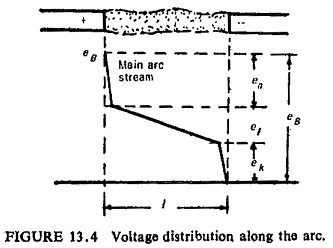The temperature of metallic arc spots is about 2000 to 3000°C, where­as with carbon electrodes the temperature rises up to 3000 to 4000°C.

The gas temperature in the are has been measured as being 5000 to 8000°C, the lower values refer to smaller currents and higher values to larger currents. True arcs with white-hot cathode, in general, are developed with currents above 0.5 A. Below this limit application of high voltage forms a glow current which does not need a hot cathode.

For moderate values of current and voltage, the Arc Properties can be expressed by Ayrton’s equation: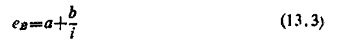Thus with rising current, the voltage decreases as a hyperbola. The constants a and b vary linearly with the length l of the arc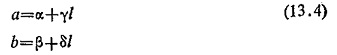Average values of α, γ, β and δ for arcs in air between copper electrodes are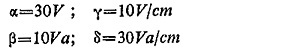The a.c. current varies periodically going through zero twice during a cycle. According to change of current the discharge alternates. If voltage and current are measured at an a.c. arc, distorted curve shapes are found depending on the type of the arc gas, the material of the electrodes and the frequency of the current.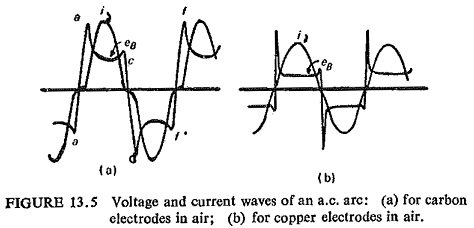Figure (13.5a) shows such curves for a carbon arc and Fig. (13.5b) for a copper arc both burning in air at normal frequency. From such changes with time the voltage-current characteristics can be directly derived as represented in Fig. (13.6a) for carbon and Fig. (13.6b) for copper electrodes.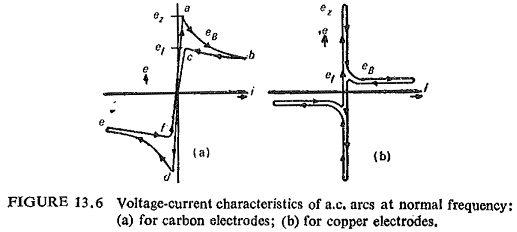These characteristics are no longer unique but consist of two different branches, one for increasing and the other for decreasing current. This difference as pointed out earlier is due to heat capacity of the electrodes and the arc gas, which are not able to follow the instantaneous condition with rapid variation of the state. Because of the great heat conduction of metal electrodes the two branches of the characteristic of Fig. (13.6b) are not so widely different as those of Fig. (13.6a) for carbon electrodes.

At points a and d arc discharges occur, at points c and f the arc is extinguished. During the intervals between points “a-a”, “c-d”, “f-f” unstable discharge takes place. Interruption of the loaded circuit is always accompanied by arc discharge between the contacts of the opening apparatus. During this process a large quantity of energy (mostly in heat form) is released in the arc space. This energy can be calculated according to the following expression: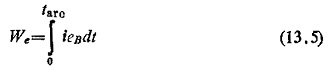where

i = instantaneous value of current

eB = voltage drop across the arc

tarc = time during which arc exists

This energy can cause damage to circuit breaker contacts, vaporization of oil, increasing of pressure inside tank, etc. Obviously by reducing the arcing time the possible damage to the circuit breaker can be avoided. In a.c. circuit breakers therefore the deionization of the Arc Properties path is easily achieved at current zero position.

It can be seen that the a.c. arc is useful in the sense that if the circuit was interrupted abruptly there would occur dangerous overvoltages between the contacts due to the inductance of the circuit. Interruption of the circuit occurs only at the instant when current reaches normal zero when the arc disappears. In other words, a.c. arc synchronizes the instant of opening of the circuit with the normal current zero irrespective of the instant of contacts parting.

Scroll to Top